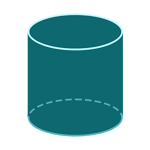Report a Problem
Suggestions

#Calculate The Volume of A Right Cylinder

Last updated: Saturday, June 24, 2023
Select a cylindrical shape below
Right Cylinder
Hollow Cylinder
Oblique Cylinder
Right Truncated
Cylindrical Wedge

A right cylinder is a three-dimensional shape that consists of two identical parallel circular bases and a curved lateral surface that connects the bases. It is also called a circular cylinder, and it is one of the most common shapes found in everyday life, from cans and bottles to pipes and tubes.

The shape of a right cylinder is found in many objects around us, from a can of soda to a smokestack. The uniform shape makes it easy to manufacture and transport products such as these, while the rounded edges of the cylinder make it easy to handle and store. The mathematical properties of the right cylinder also make it useful in engineering and architecture, where it is used in the design of columns, pillars, and beams.

Easily calculate the volume of a right cylinder with step-by-step guidance using our free online calculator below.

The formula for determining the volume of a right cylinder is defined as:
$$V$$ $$=$$ $$\pi$$ $$\cdot$$ $$r^2$$ $$\cdot$$ $$h$$
$$V$$: the volume of the cylinder
$$r$$: the radius of the base
$$h$$: the height of the cylinder
The SI unit of volume is: $$cubic \text{ } meter\text{ }(m^3)$$

## Find $$V$$

Use this calculator to determine the volume of a cylinder when the radius of the bases and the height are given.
Hold & Drag
CLOSE
$$r$$
$$meter$$
$$h$$
$$meter$$